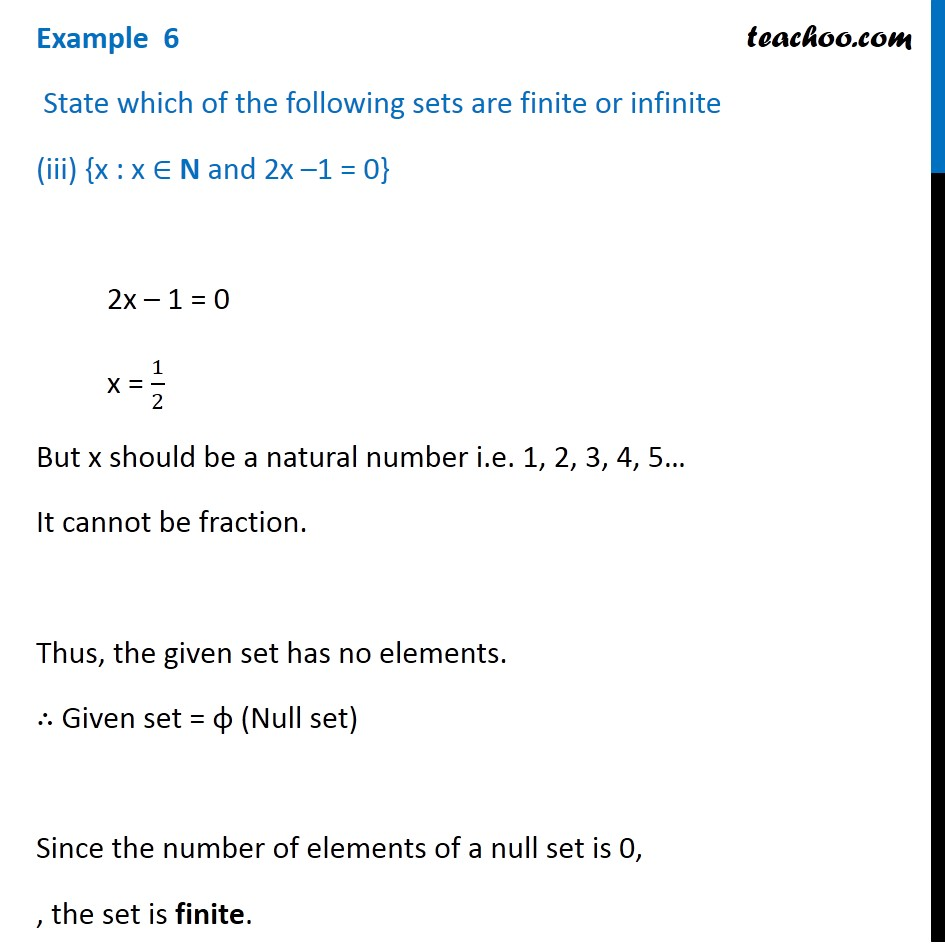Examples

Chapter 1 Class 11 Sets
Serial order wiseGet live Maths 1-on-1 Classs - Class 6 to 12

### Transcript

Example 6 State which of the following sets are finite or infinite (iii) {x : x ∈ N and 2x –1 = 0} 2x – 1 = 0 x = 1/2 But x should be a natural number i.e. 1, 2, 3, 4, 5… It cannot be fraction. Thus, the given set has no elements. ∴ Given set = φ (Null set) Since the number of elements of a null set is 0, , the set is finite.